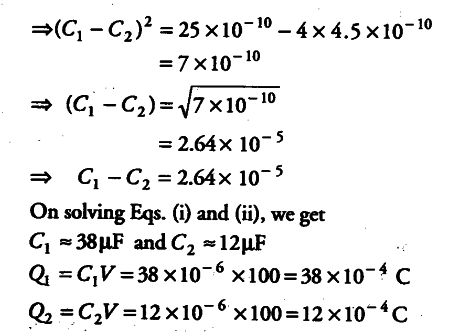# Two capacitors of unknown capacitances

Two capacitors of unknown capacitances \${ C }{ 1 }\$ and
\${ C }
{ 2}\$ are connected first in series and then in parallel across a battery of 100 V.
If the energy stored in the two combinations is 0.045 J and 0.25 J respectively, then determine the value of \${ C }{ 1 }\$
and \${ C }
{ 2}\$ Also, calculate the charge on each capacitor in parallel combination.

When the capacitors are connected in parallel, equivalent capacitance,